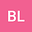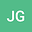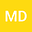The state-of-the-art modeling of cosmogenic Cr isotopes produced in lunar rocks compared with existing calculations and measurements
•••• +3Jia Liu
University of Science and Technology of China, Hefei 230026, P.R. China
Author ProfileYingnan Zhang
Environment Research Institute, Shandong University
Author ProfileLiping Qin
University of Science and Technology of China
Author Profile## Abstract

The distribution of Cr isotopes provides useful information to trace the source and origin of extraterrestrial samples, but it is usually influenced by high-energy cosmic rays. Since lunar and terrestrial materials have quite similar Cr isotope compositions, distinguishing the effect of cosmic rays in lunar samples is especially important. Those cosmic radiation particles (primary particles) can react with lunar materials, creating many secondary particles. Both primary and secondary particles can produce cosmogenic nuclides on the Moon. Radiation Environment and Dose at the Moon (REDMoon) is a novel GEANT4 Monte-Carlo model built to simulate the interactions of space particles with the lunar surface and subsurface content. Using this model, we simulate the production of cosmogenic Cr isotopes ($^{50}$Cr,$^{52}$Cr,$^{53}$Cr,$^{54}$Cr) at different depths of lunar surface, and compare the contribution of different reactions generating these nuclides. The results suggest that spallation reactions are the most important process producing cosmogenic Cr isotopes. We also analyze the relationship between $^{53}$Cr/$^{52}$Cr and $^{54}$Cr/$^{52}$Cr predicted by our model and compare it with different Apollo samples. As previously studied, we also find an approximate linear relationship between $\varepsilon^{53}$Cr and $\varepsilon^{54}$Cr (per 10,000 deviation of $^{53}$Cr/$^{52}$Cr and $^{54}$Cr/$^{52}$Cr ratios from the standard). Furthermore, we reveal a change of this linear relationship in different depths of lunar surface. Besides, we investigate how the slopes can be influenced by exposure age and the Fe/Cr ratio. With these additional factors carefully considered, the comparison between our modeled results and the measurements is better than previous studies.
30 Aug 2023Submitted to ESS Open Archive
11 Sep 2023Published in ESS Open Archive